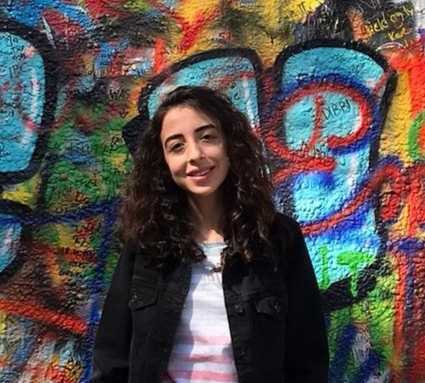Knowledge&Technology

# Applications of Hashing Algorithms on Images

In this article, we will apply various hash algorithms to images. Well, what is this hash? I seem to hear you say, if I say Cryptology as a clue, maybe it can give you an idea. As you know, Cryptology is the science of cryptography.Ezgisu Akat
2 minutes

# Applications of Hashing Algorithms on Images

In this article, we will apply various hash algorithms to images. Well, what is this hash? I seem to hear you say, if I say Cryptology as a clue, maybe it can give you an idea. As you know, Cryptology is the science of cryptography.

What about hash? Let's make our explanations without making you wait any longer. Hashing is a hash function, compressing the message it receives into a smaller space. The hash function produces data outputs that cannot be recycled. In other words, unlike the encryption process, the original data cannot be recovered in the hashing process. As examples of hashing algorithms, we can give MD5, SHA.

For example, the MD5 function is an algorithm that reduces the message it receives to 128 bits. In this article, we will create hash values for our images. So why? We can create a hash value in our images and store this hash value in the database instead of the image. Instead of storing the pictures in the database, we can keep the hash of the pictures. And in this way, we can access the data faster in the database. But in this article, we will measure the similarity ratio between the pictures by keeping the hash information of the pictures.

We will apply average hashing, difference hashing, perceptual hashing, wavelet hashing, HSV color hashing, and crop-resistant hashing algorithms on our pictures, respectively.

The second image is the gray armchair.

If you are ready, we can start by making our installations. I used Python 3.10.5 version, pillow and Imagehash library, and Pycharm as IDE.

LINE 2-3: First, we added our pillow and image hash package to our project. The hash functions we use in the Imagehash library consist of 64 bits as output to us.

LINE 6: The open function of the Image module reads and uploads our first image file.

LINE 7: The average_hash function of the imagehash module produces the hash value of our image as a parameter. The average hash algorithm scales down the input image after first converting it to grayscale. In this situation, the image is reduced to 8x8 pixels because we want to create a 64-bit hash. The most straightforward algorithm, average hashing, involves just a few transformations. Also, the average hash for each of the pixels outputs 1 if the pixel is bigger or equal to the average and 0 otherwise.

LINE 10-11-12: The same operations were performed on the first image, and the average hash was applied to the second image.

LINE 14: We measure how similar the pictures are by taking the difference in the average hashing values obtained for the two pictures. The difference in hash values of these two images is 19. The smaller this difference value, the more similar it is. Actually, we can say that these two images have a hamming distance of 10.

Now let's do difference hashing.

LINE 17-19: Here, we applied the difference hashing algorithm to our images using the dhash function. The difference hash algorithm, like the average hash algorithm, first converts the input image to a grayscale image, which is then scaled down to 9x8 pixels. Similar to the typical hash technique, the first 8 pixels from each row are evaluated serially from left to right and compared to their neighbor to the right, producing a 64-bit hash.

LINE 22: The difference in hashing values we obtained with the dhash function we applied to our images is 23.

LINE 25-27: We applied a perceptual hashing algorithm to our images with the phash function. Perceptive hash does the same as aHash, but first, it does a Discrete Cosine Transformation and works in the frequency domain. The perceptual hash algorithm also originally calculated and reduced the gray value image.

LINE 29: Again, we found the hash value difference between the two images to be 28.

LINE 32-34: We implemented the wavelet hashing algorithm using the whash function. The wavelet hashing works in the frequency domain as pHash, but it uses DWT instead of DCT. The wavelet hash algorithm creates an 8x8 gray value image, much like the average hash method does. The image is then given a two-dimensional wavelet transform. Our experiments have shown that the results are improved when the top row is set to 0, or black, and the wavelet transform is applied three more times.

LINE 37: We found the wavelet hashing difference of 12 between the two images.

LINE 40-42: This time we applied hsv color hashing to our images using the colorhash function. Hsv color hashing computes fractions of the image in intensity, hue, and saturation bins.

LINE 45: The difference between the hash values of these two colorhash images is 8.

Finally, let's do crop resistant hashing.

LINE 48-50: We applied the crop resistant hashing algorithm to our images by using the crop_resistant_hash function of the imagehash module. Using a watershed-like algorithm, this algorithm divides the image into bright and dark segments before performing an image hash on each segment. Since most other algorithms stop at roughly 5% cropping, this makes the image far more resistant to cropping than other algorithms. The study claims resistance to up to 50% cropping.

LINE 51: The difference in the hash values of our images for which we applied the crop resistant hashing algorithm 4.

And now we have come to the end of our article, I hope you enjoyed reading it.